International
Tables for
Crystallography
Volume B
Reciprocal space
Edited by U. Shmueli

International Tables for Crystallography (2010). Vol. B, ch. 1.3, p. 89   | 1 | 2 |

## Section 1.3.4.3.6.2. Monoclinic groups

G. Bricognea

aGlobal Phasing Ltd, Sheraton House, Suites 14–16, Castle Park, Cambridge CB3 0AX, England, and LURE, Bâtiment 209D, Université Paris-Sud, 91405 Orsay, France

#### 1.3.4.3.6.2. Monoclinic groups

| top | pdf |

A general monoclinic transformation is of the form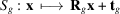witha diagonal matrix whose entries areor, anda vector whose entries are 0 or. We may thus decompose both real and reciprocal space into a direct sum of a subspacewhereacts as the identity, and a subspacewhereacts as minus the identity, with. All usual entities may be correspondingly written as direct sums, for instance: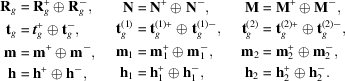We will use factoring by 2, with decimation in frequency when computing structure factors, and decimation in time when computing electron densities; this corresponds towith,. The nonprimitive translation vectorthen belongs to, and thusThe symmetry relations obeyed byand F are as follows: for electron densitiesor, after factoring by 2,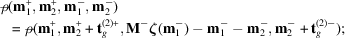while for structure factorswith its Friedel counterpartor, after factoring by 2,with Friedel counterpartWhen calculating electron densities, two methods may be used.

 (i) Transform onfirst. The partial vectors defined by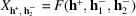obey symmetry relations of the formwithindependent of. This is the same relation as for the same parity class of data for a (complex or real) symmetricor antisymmetrictransform. The same techniques can be used to decrease the number ofby multiplexing pairs of such vectors and demultiplexing their transforms. Partial vectors with different values ofmay be mixed in this way (Section 1.3.4.3.5.6). Onceis completed, its results have Hermitian symmetry with respect to, and the methods of Section 1.3.4.3.5.1may be used to obtain the unique electron densities. (ii) Transform onfirst. The partial vectors defined by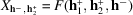obey symmetry relations of the formwithindependent of. This is the same relation as for the same parity class of data for a Hermitian symmetricor antisymmetrictransform. The same techniques may be used to decrease the number of. This generalizes the procedure described by Ten Eyck (1973)for treating dyad axes, i.e. for the case, and(simple dyad) or(screw dyad). Onceis completed, its results have Hermitian symmetry properties with respect towhich can be used to obtain the unique electron densities. Structure factors may be computed by applying the reverse procedures in the reverse order.

### References

Ten Eyck, L. F. (1973). Crystallographic fast Fourier transforms. Acta Cryst. A29, 183–191.